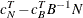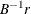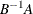### Output Data Sets

The LP procedure can optionally produce five output data sets. These are the ACTIVEOUT=, PRIMALOUT=, DUALOUT=, TABLEAUOUT=, and MPSOUT= data sets. Each contains two variables that identify the particular problem in the input data set. These variables are

`_OBJ_ID_`

identifies the objective function ID.

`_RHS_ID_`

identifies the right-hand-side variable.

Additionally, each data set contains other variables, which are discussed below.

#### ACTIVEOUT= Data Set

The ACTIVEOUT= data set contains a representation of the current active branch-and-bound tree. You can use this data set to initialize the branch-and-bound tree to continue iterations on an incompletely solved problem. Each active node in the tree generates two observations in this data set. The first is a 'LOWERBD' observation that is used to reconstruct the lower-bound constraints on the currently described active node. The second is an 'UPPERBD' observation that is used to reconstruct the upper-bound constraints on the currently described active node. In addition to these, an observation that describes the current best integer solution is included. The data set contains the following variables:

`_STATUS_`

contains the keywords LOWERBD, UPPERBD, and INTBEST for identifying the type of observation.

`_PROB_`

contains the problem number for the current observation.

`_OBJECT_`

contains the objective value of the parent problem that generated the current observation’s problem.

`_SINFEA_`

contains the sum of the integer infeasibilities of the current observation’s problem.

`_PROJEC_`

contains the data needed for CANSELECT=PROJECT when the branch-and-bound tree is read using the ACTIVEIN= option.

`_PSEUDO_`

contains the data needed for CANSELECT=PSEUDOC when the branch-and-bound tree is read using the ACTIVEIN= option.

`INTEGER VARIABLES`

Integer-constrained structural variables are also included in the ACTIVEOUT= data set. For each observation, these variables contain values for defining the active node in the branch-and-bound tree.

#### PRIMALOUT= Data Set

The PRIMALOUT= data set contains the current primal solution. If the problem has integer-constrained variables, the PRIMALOUT= data set contains the current best integer feasible solution. If none have been found, the PRIMALOUT= data set contains the relaxed solution. In addition to `_OBJ_ID_` and `_RHS_ID_`, the data set contains the following variables:

`_VAR_`

identifies the variable name.

`_TYPE_`

identifies the type of the variable as specified in the input data set. Artificial variables are labeled as type 'ARTIFCL'.

`_STATUS_`

identifies whether the variable is basic, nonbasic, or at an upper bound in the current solution.

`_LBOUND_`

contains the input lower bound on the variable unless the variable is integer-constrained and an integer solution is given. In this case, `_LBOUND_` contains the lower bound on the variable needed to realize the integer solution on subsequent calls to PROC LP when using the PRIMALIN= option.

`_VALUE_`

identifies the value of the variable in the current solution or the current best integer feasible solution.

`_UBOUND_`

contains the input upper bound on the variable unless the variable is integer-constrained and an integer solution is given. In this case, `_UBOUND_` contains the upper bound on the variable needed to realize the integer solution on subsequent calls to PROC LP when using the PRIMALIN= option.

`_PRICE_`

contains the input price coefficient of the variable.

`_R_COST_`

identifies the value of the reduced cost in the current solution. Example 6.3 in the section Examples: LP Procedure shows a typical PRIMALOUT= data set. Note that it is necessary to include the information on objective function and right-hand side in order to distinguish problems in multiple problem data sets.

#### DUALOUT= Data Set

The DUALOUT= data set contains the dual solution for the current solution. If the problem has integer-constrained variables, the DUALOUT= data set contains the dual for the current best integer solution, if any. Otherwise it contains the dual for the relaxed solution. In addition to `_OBJ_ID_` and `_RHS_ID_`, it contains the following variables:

`_ROW_ID_`

identifies the row or constraint name.

`_TYPE_`

identifies the type of the row as specified in the input data set.

`_RHS_`

gives the value of the right-hand side on input.

`_L_RHS_`

gives the lower bound for the row evaluated from the input right-hand-side value, the TYPE of the row, and the value of the RANGE variable for the row.

`_VALUE_`

gives the value of the row, at optimality, excluding logical variables.

`_U_RHS_`

gives the upper bound for the row evaluated from the input right-hand-side value, the TYPE of the row, and the value of the RANGE variable for the row.

`_DUAL_`

gives the value of the dual variable associated with the row.

#### TABLEAUOUT= Data Set

The TABLEAUOUT= data set contains the current tableau. Each observation, except for the first, corresponds to a basic variable in the solution. The observation labeled `R_COSTS` contains the reduced costs. In addition to `_OBJ_ID_` and `_RHS_ID_`, it contains the following variables:

`_BASIC_`

gives the names of the basic variables in the solution.

`INVB_R`

gives the values of, whereis the right-hand-side vector.

`STRUCTURAL VARIABLES`

give the values in the tableau, namely.

#### MPSOUT= Data Set

The MPSOUT= data set contains problem data converted from a PROC LP format into an MPS-format SAS data set. The six fields, `FIELD1` to `FIELD6`, in the MPSOUT= data set correspond to the six columns in MPS standard. For more information about the MPS-format SAS data set, see Chapter 15: The MPS-Format SAS Data Set in SAS/OR 12.1 User's Guide: Mathematical Programming.